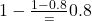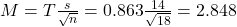## A botanist wishes to estimate the mean number of seeds for a certain fruit. She samples 18 specimens and finds the average number of seeds i

Question

A botanist wishes to estimate the mean number of seeds for a certain fruit. She samples 18 specimens and finds the average number of seeds is 48 with a standard deviation of 14. Construct a one-sided upper-bound 80% confidence interval for the mean number of seeds for the species using the appropriate critical value to three decimal places.

in progress 0
5 months 2021-08-25T07:43:17+00:00 1 Answers 0 views 0

The 80% confidence interval for the mean number of seeds for the species is of 50.848 seeds.

Step-by-step explanation:

We have the standard deviation for the sample, which means that the t-distribution is used to solve this question.

T interval

The first step to solve this problem is finding how many degrees of freedom, we have. This is the sample size subtracted by 1. So

df = 18 – 1 = 17

80% confidence interval

Now, we have to find a value of T, which is found looking at the t table, with 17 degrees of freedom(y-axis) and a confidence level(as we want the one-sided upper-bound confidence interval) of. So we have T = 0.863

The margin of error is:In which s is the standard deviation of the sample and n is the size of the sample.

The upper end of the interval is the sample mean added to M. So it is 48 + 2.848 = 50.848 seeds.

The 80% confidence interval for the mean number of seeds for the species is of 50.848 seeds.